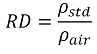# Relative Density Calculation (based on Standard Density)

### Relative Density Calculation (based on Standard Density)

#### This method is used to calculate the relative density from the standard density

Relative density is calculated as follows:Symbol Description Units
RD Relative density -
ρstd Standard density kg/Sm3

Constant Description Value Units
ρair Standard density of dry air 1.225410 kg/Sm3